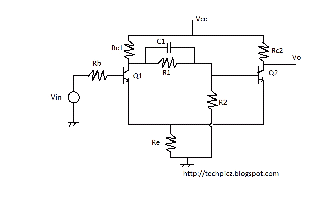## Monday, 9 July 2012

### SCHMITT TRIGGER CIRCUIT USING BC107

﻿The schmitt trigger is an emitter coupled bistable multivibrator with its cross-coupling is removed . It is comparator that is used to convert a periodical random analog wave to square wave having the same frequency of the analog wave. Due to this schmitt trigger is called a squaring circuit.
In this circuit diagram output of this circuit goes high level whan the amplitude of the input goes have above a predetermined level called upper treshold point(utp) output of this circuit goes low level when the amplitude of the input signal goes below a predetermined level called lower treshold point (ltp). The schmitt trigger compares the input analog wave form  with respect to the preset values of UTP and LTP . So it is known as two level comparator.
Without any input signal , transistor Q1 stays in state and Q2 in saturation state. Current Ie2 flows trougth the common emmiter resistor Re causing a potential drop equal to Ie2Re. This point called UTP. When the input amplitude increase and reaches UTP Q1 turns ON. Subsequently Q2 turns OFF and the output raises to Vcc. Now current Ie2 become zero and Ie2 starts flowing through Re. The minimum voltage required to hold the transistor Q1 ON is equal to Vbe cut in + Ie1Re1. When the amplitude of the input sinwave become lessthan this, Q1 turns off, in turns Q2 turns on and the output voltage drops. The value of the input voltage at which transistor Q2 turns on is called LTP.
components for wiring this circuit
Q1 = Q2= BC107
Rb = 47K
Rc1 = 4.7K
Rc2 = 1.5K
R1 = 10K
R2 = 33K
Re = 3.3K
C1 = 4.7pF
Vin = 20Vpp
Vcc = 8V
Vo= output